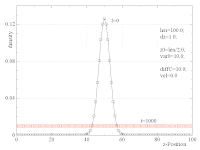## Friday, May 22, 2009

### Cell invasion (4) : Numerical solution of drift-diffusion equation

Back

The cell invasion problem requires an accurate numerical solution of coupled partial differential equations. The fields (concentration profiles) f(z,t) will depend on one spatial coordinate z and the time t. The spatial coordinates will be restricted to the intervall [0,len], with well-defined boundary conditions at the left and right limits: The current must dissapear outside the intervall.

I will try to solve this problem by discretizing the z-coordinate into a regular grid,
z_k = k\;\Delta z,

and then treating each z_k as a separate dynamical variable. The problem can thereby be mapped onto a set of coupled ordinary differential equations, which are solved with the Runga-Kutta method.

As a simple test and practice case, consider the drift-diffusion equation
\frac{\partial}{\partial t}c(z,t) = - v \frac{\partial}{\partial z}c(z,t) + D \frac{\partial^2}{\partial z^2}c(z,t),

with position- and time-independent diffusion constant D and drift velocity v.

For the following tests, we set the initial distribution c(z,t) equal to a narrow, normalized Gaussian (variance=10), placed at the center of the intervall. The spatial resolution is set to dz=1.

Test 1: Free diffusion in a box

First we set the drift-velocity equal zero. The initial peak should broaden with time. Asymptotically, a flat distribution should develop, while the integral (total particle number) is preserved. The simulation shows the expected behaviour:Test 2: Diffusion with drift (boundaries far away)

The case of combined diffusion and drift has been compared directly with the analytical solution (solid lines). One expects the center z_c(t) of the Gaussian to move according to
z_c(t) = v\;t

and the variance to grow linearly
\sigma^2(t)=\sigma^2(0)+ 2Dt

The simulation (dashed lines) fits the analytical curves nicely, even for the modest spatial resolution of dz=1:Test 3: Diffusion with drift against a wall

Finally, we choose a negative drift velocity and wait until the distribution is pushed againts the left wall. We now expect the stationary solution
c(z)=\frac{v}{D}\;e^{-(v/D)z}.

Again, the simulation (symbols) agrees very well with the analytical solution (solid lines):As a conclusion, the simple numerical method seems to work as expected. I have also checked that the results do not change significantly when the resolution dz is reduced.

Next

1.Hello Dr. Metzner.
I am a student of Physics at the Indian Institute of Science Education & Research, Kolkata.
I have been studying the Smoluchowski convection-diffusion equation for some time and wish to try it out hands on. I have proceeded a bit with a simple finite difference integration scheme which is very coarse.
If you can send me the Runge-Kutta code at abhranil[at]abhranil[dot]net, I can adapt it into python and learn a bit about both the equation and how RK offers advantages. I will be happy to share the results in my own blog and let you know. I do not intend to do any serious work on it, but if I do, I assure you that due credit shall be given for your code.
Thanks a lot!

1.Hello,

thanks for your comment. Here is some example code in C++ how to solve a coupled set of ordinary differential equations with Runge-Kutta forward integration:

https://dl.dropboxusercontent.com/u/1720979/blogMaterial/GMD.zip

The example is not diffusion, but two bodies in 3D with gravitational attraction. The central Runge-Kutta routine is called "DoContinuousChanges" in the main program "GMD.cpp".

Have fun,
Claus.